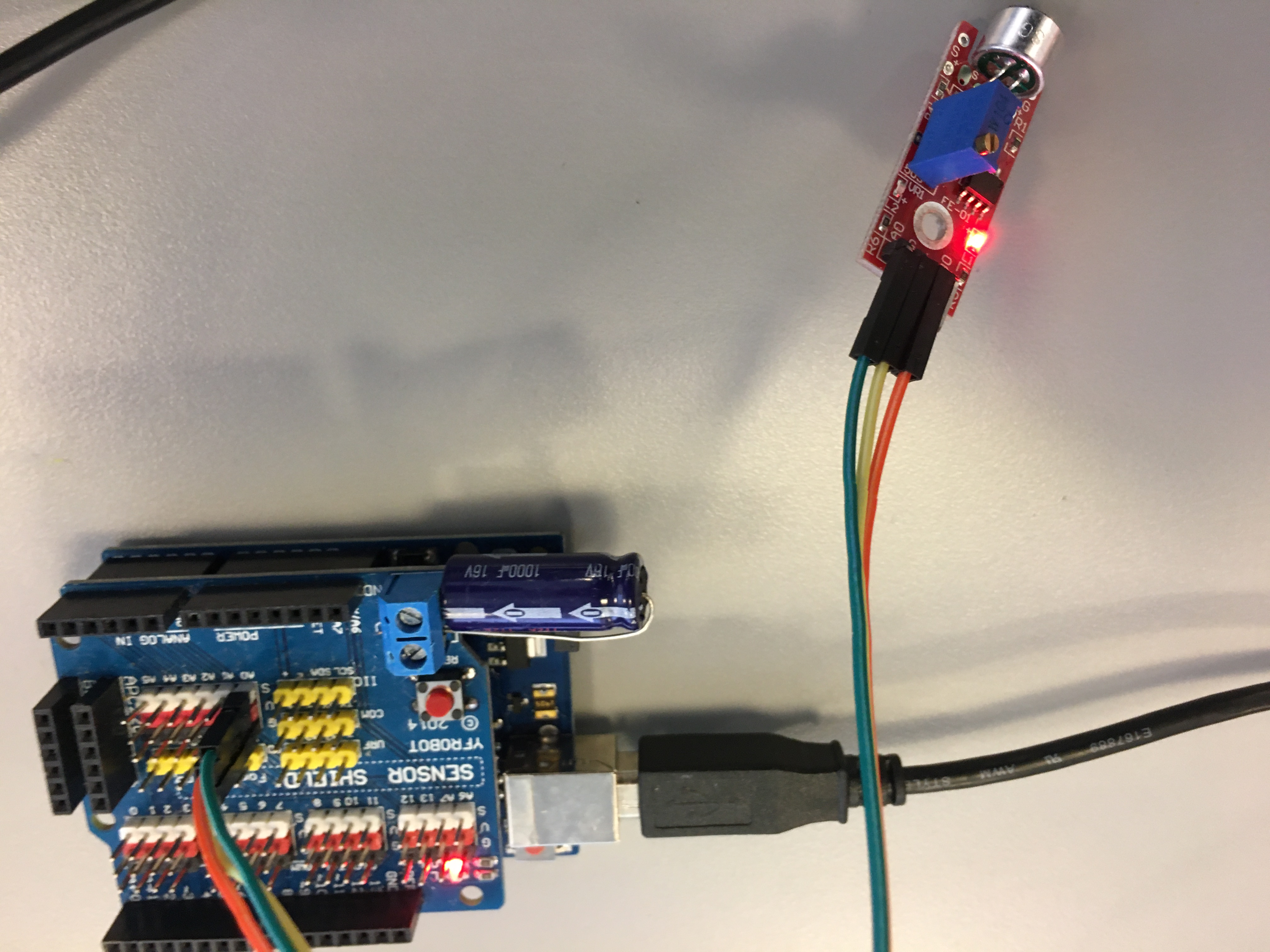Published: February 14, 2017, Edited by: Mads Hobye

# Maker kit tutorial

This is an in progress tutorial

The IO kit is a collection of components that enables you to make a wide array of experiments. The kit is made for interactive design module 2 for Humtek.

### Box design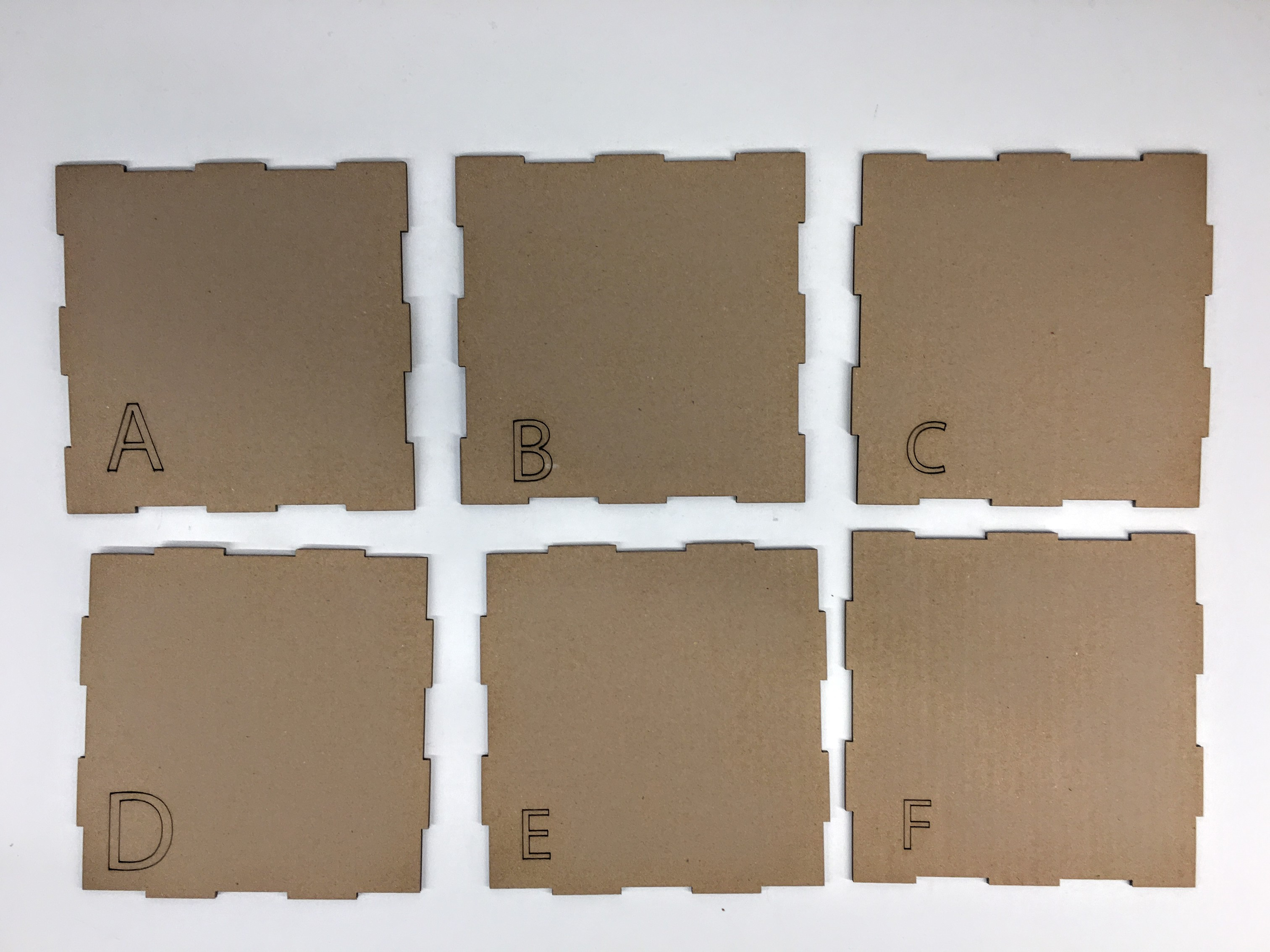### Components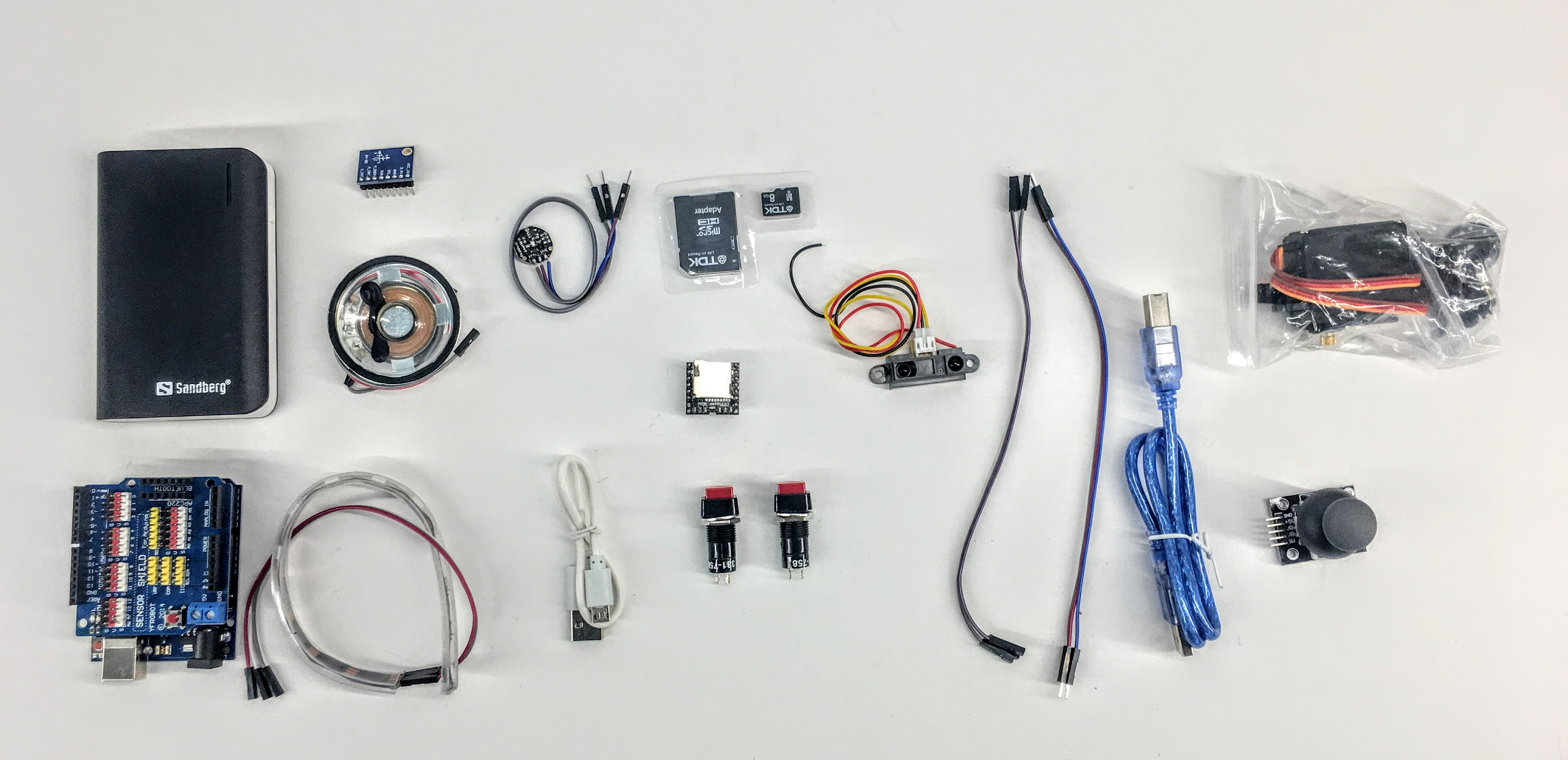• Powerbank
• Micro usb cable for power bank
• 2xButtons
• SDCard
• Micro SD card converter
• USB Cable
• Arduino With Shield
• Joystick
• Neopixel string
• Infrared Distance sensor.
• MP3 Player
• IMU
• Servo motor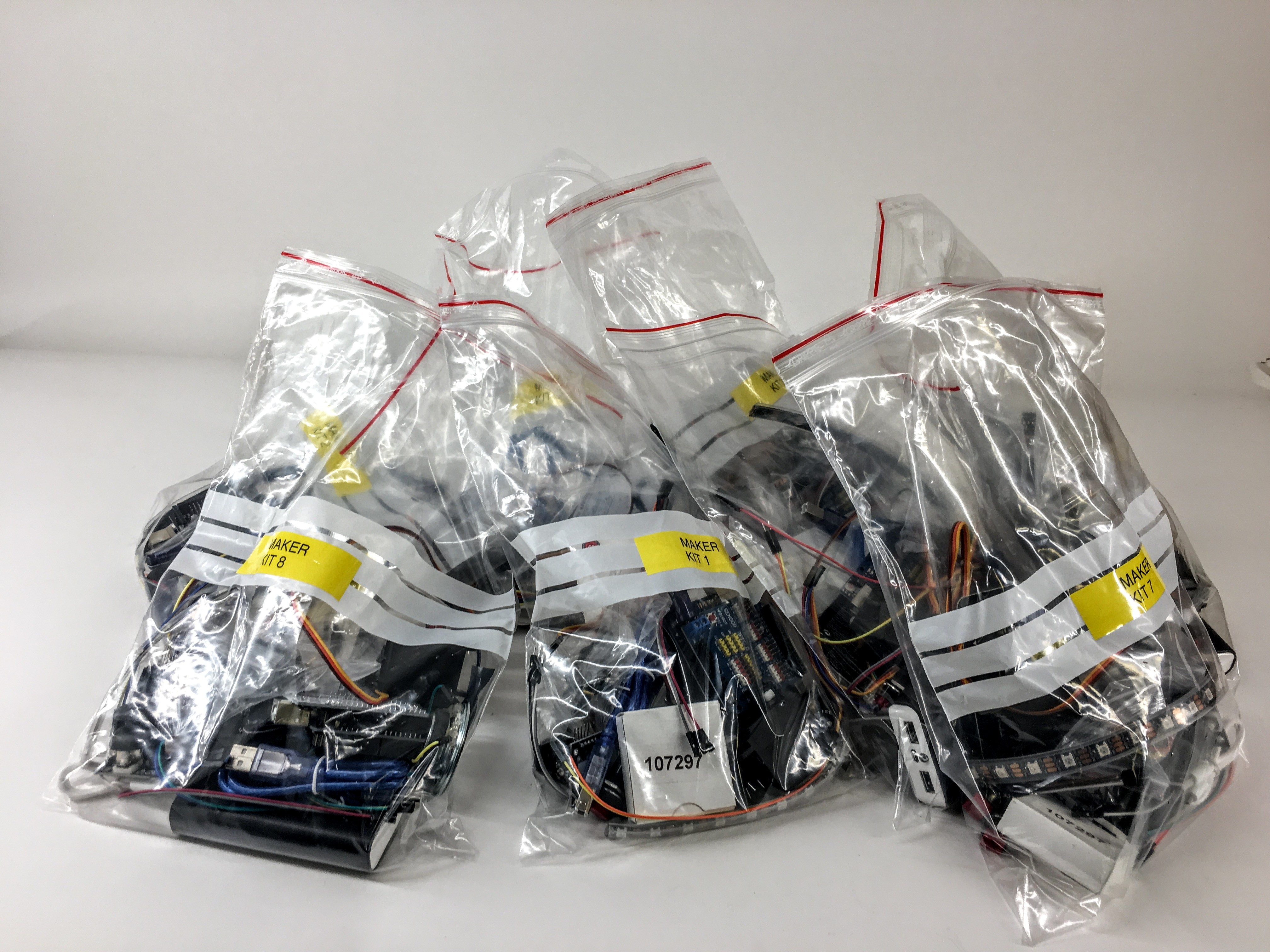## Create a touch sensor

The simplest and most interesting input is a touch sensor. Do the following:

Go to manage libraries: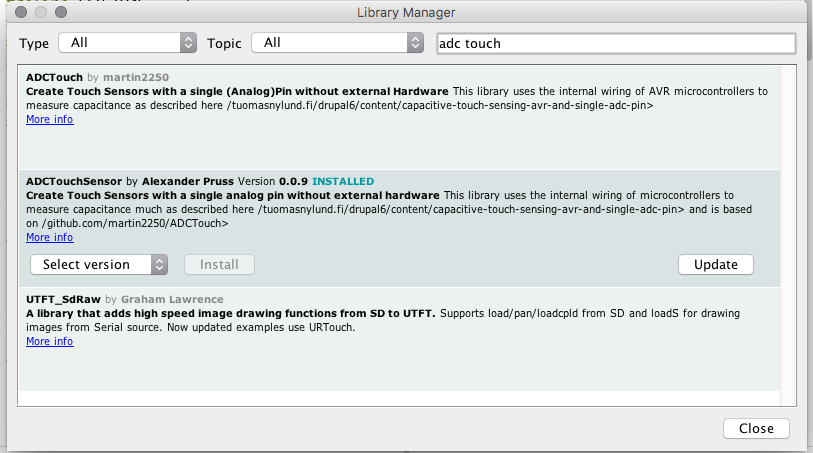Put one wire in analog zero.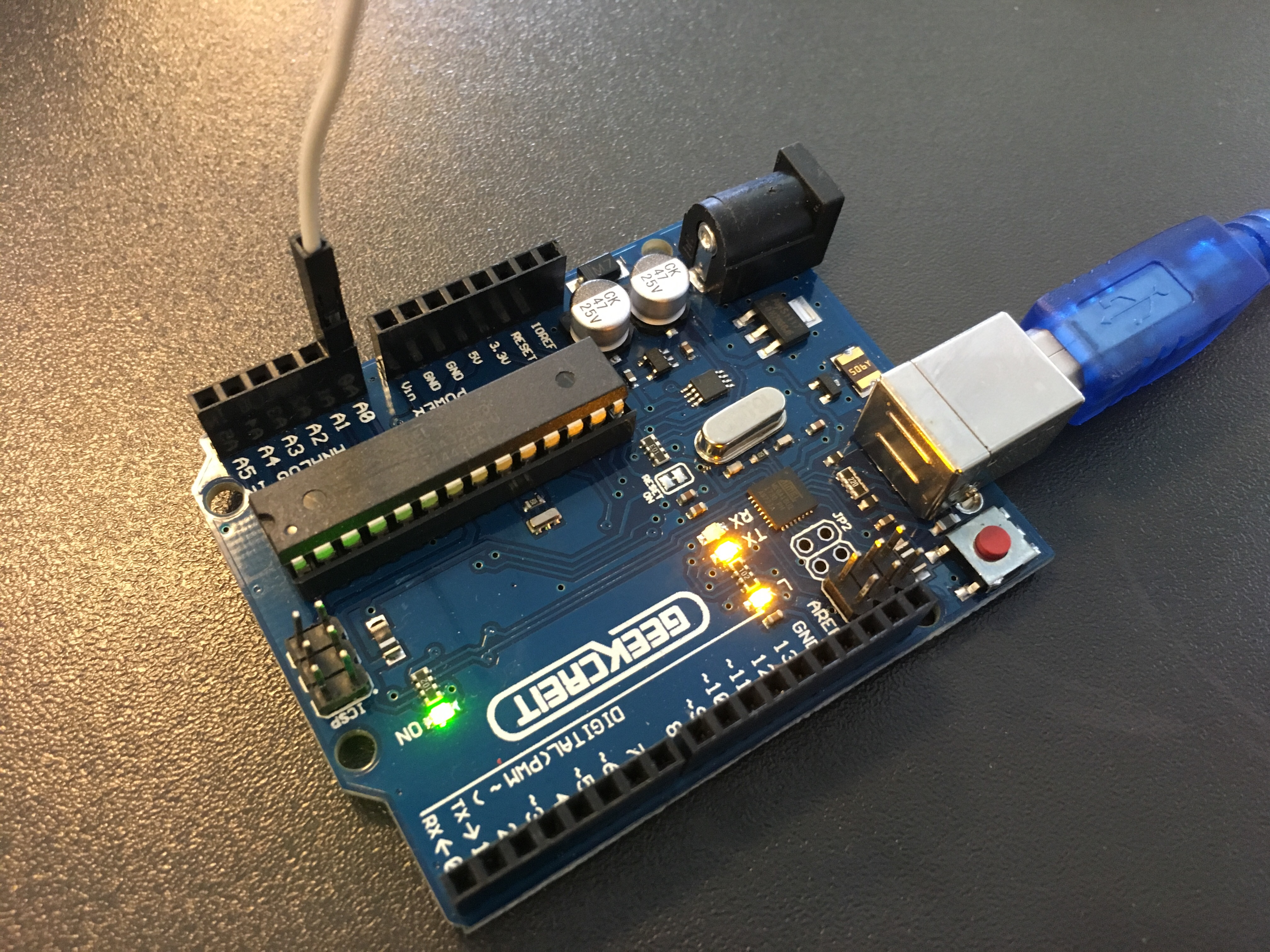Use the following code:

``````    #include <ADCTouchSensor.h>

void setup()
{
Serial.begin(9600);

touch0.begin();

}

void loop()
{
Serial.println(value0);    //send (boolean) pressed or not pressed

}
``````

Go to the serial monitor to see the output (top left corner):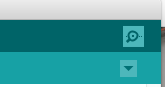## Detecting button pressed STATE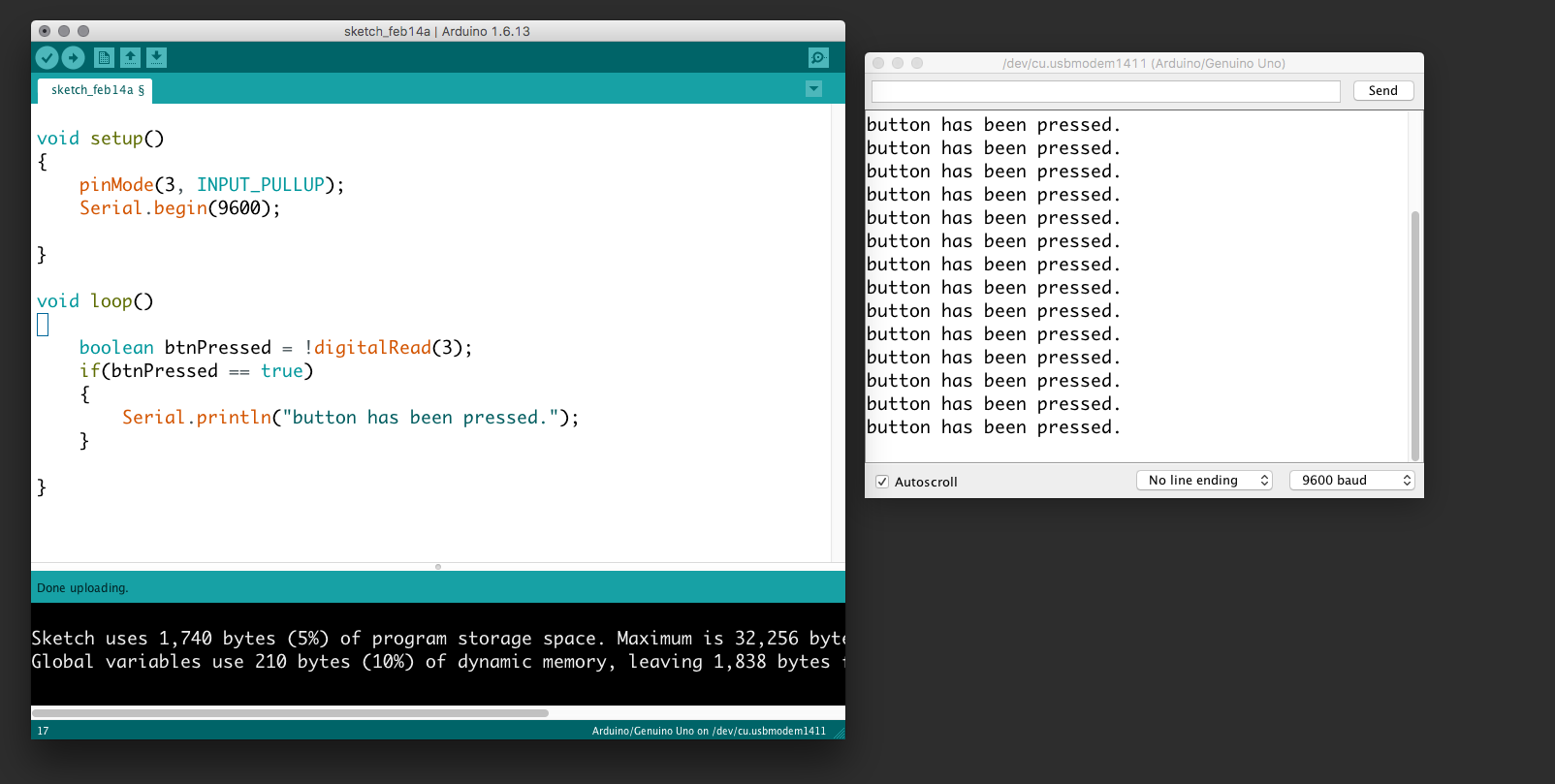In the following example, we connect a button to the Arduino and send a message repeatiatly somebody presses it. Be aware that we use the internal pull-up so the button has to be connected to the ground. Further, we use the serial communication to receive the messages to our prompt. Use the "search icon" (upper right corner) to view the serial prompt.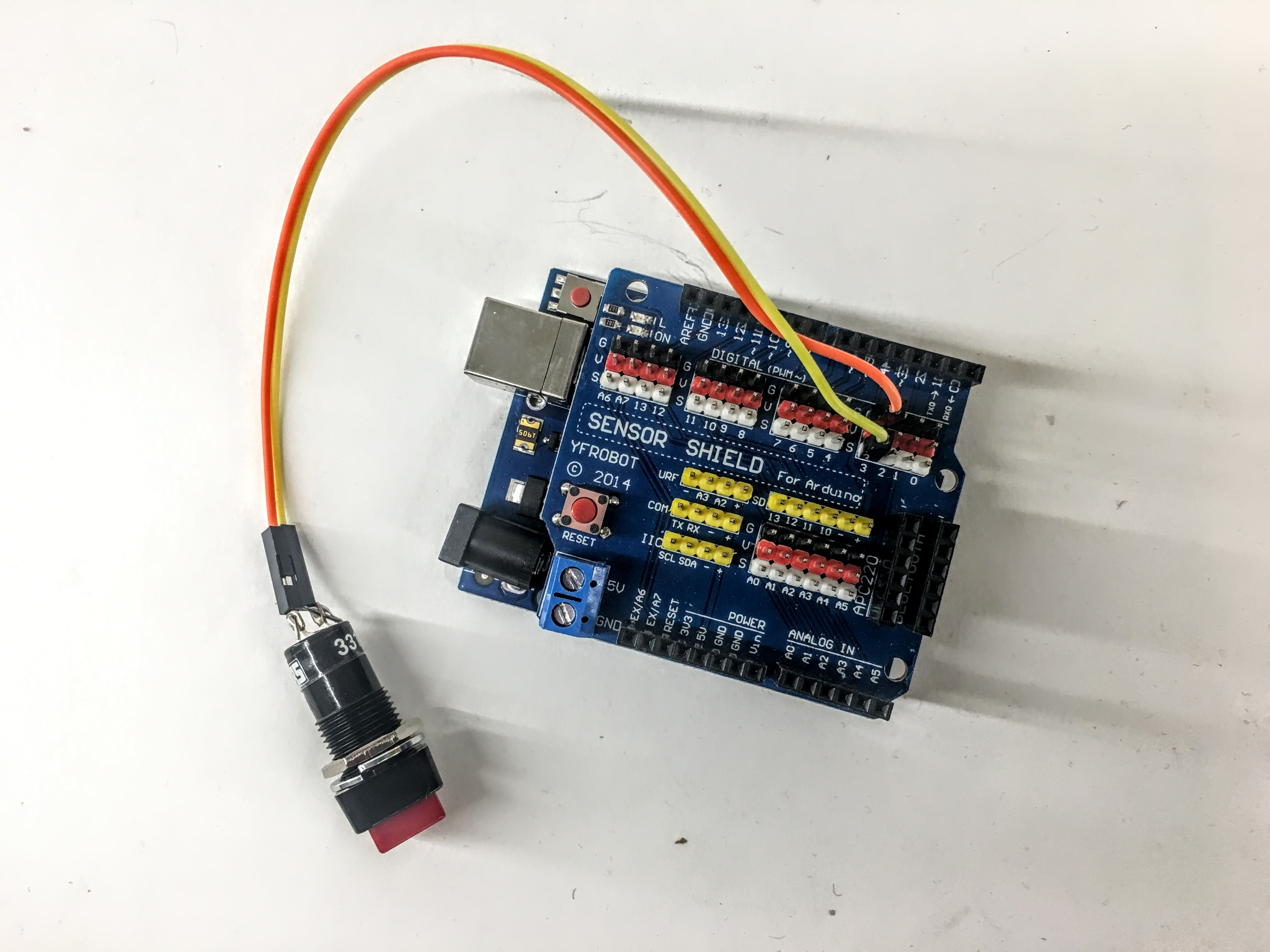``````        void setup()
{
pinMode(3, INPUT_PULLUP);
Serial.begin(9600);

}

void loop()
{
if(btnPressed == true)
{
Serial.println("button has been pressed.");
}

}
``````

## Detecting button single press - An EVENT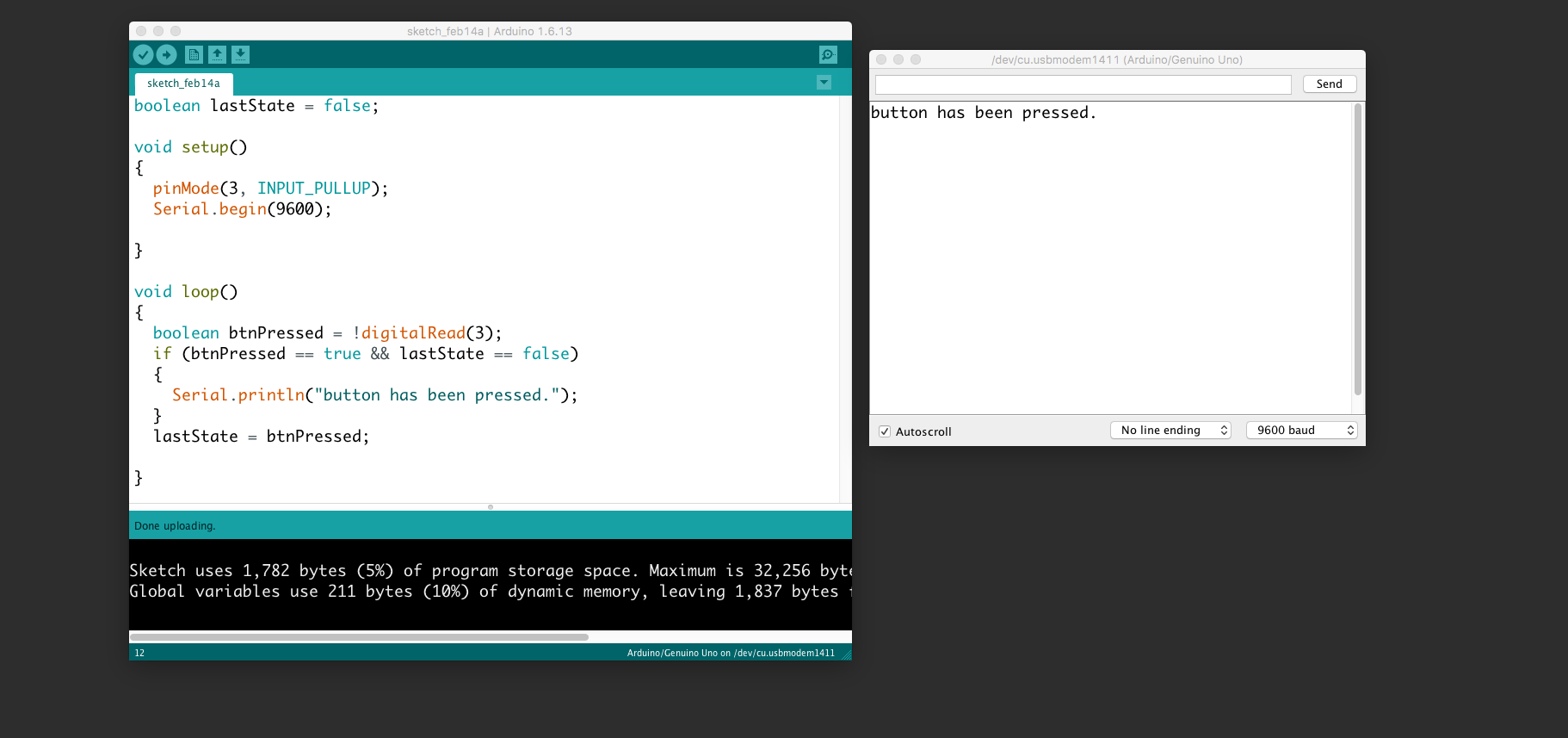The event of pressing the button. Use the same wiring as above.

``````  boolean lastState = false;

void setup()
{
pinMode(3, INPUT_PULLUP);
Serial.begin(9600);

}

void loop()
{
if (btnPressed == true && lastState == false)
{
Serial.println("button has been pressed.");
}
lastState = btnPressed;

}
``````

## Control neopixels

Neopixels are versatile light strings where each diode can be controlled individually. Each one has Red, Green, and Blue and can be from 0 to 255 in brightness. Multiple libraries exists to use noepixels. We suggest you use fastled.Search for "fastled" and click install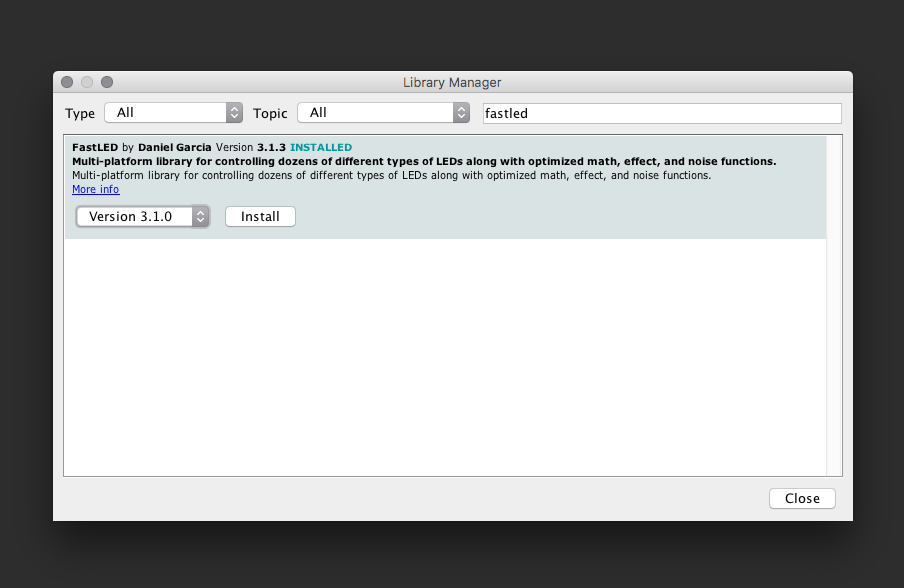Use this wiring: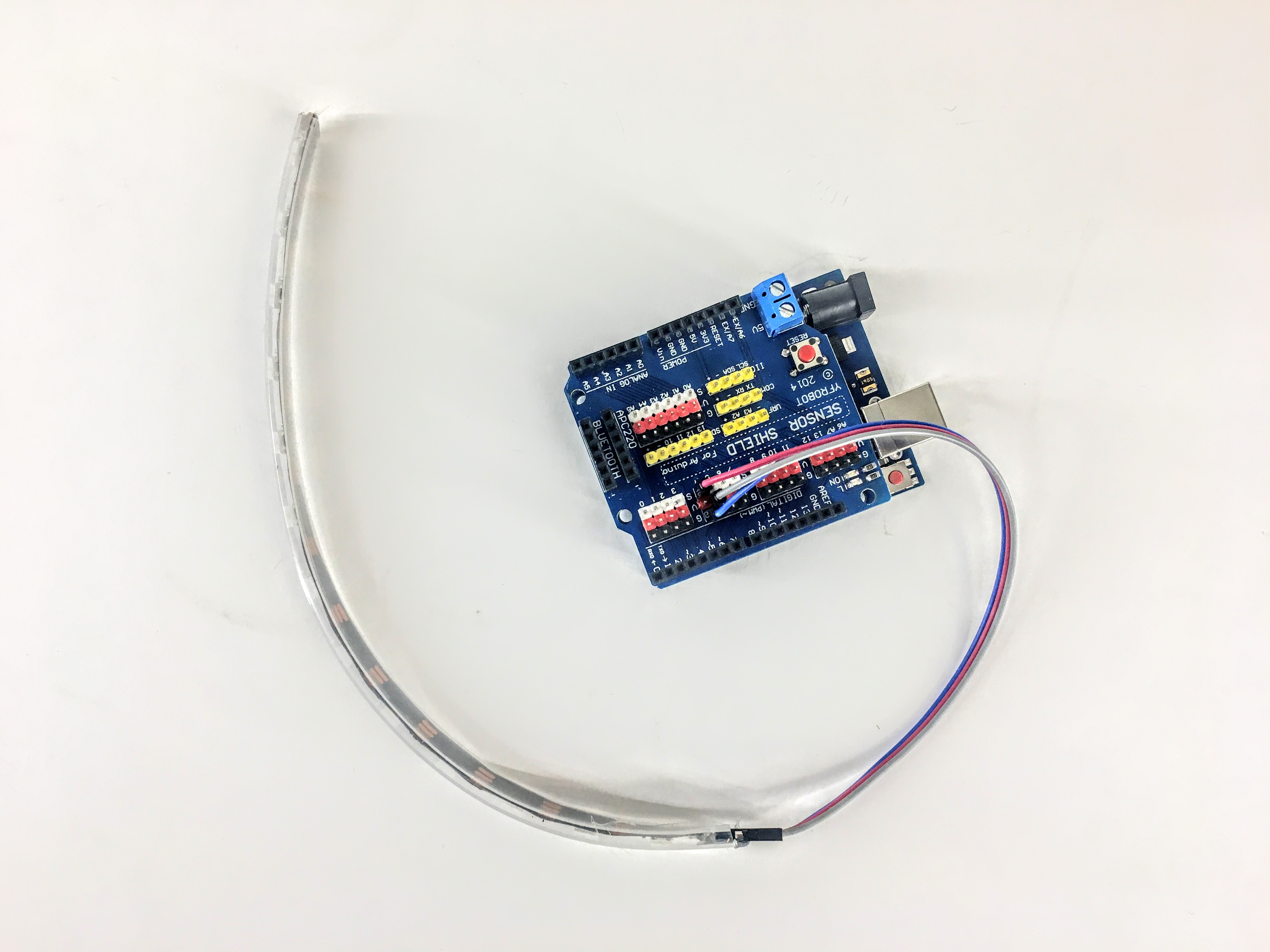IMPORTANT: connect 5v to v, Di to s and G to g AND make sure the direction is correct: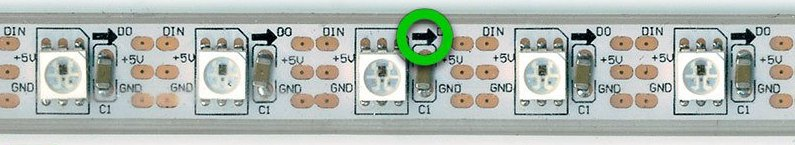This example sketch will turn red, green and blue for the first three pixels:

``````  #include "FastLED.h"

// How many leds in your strip?
#define NUM_LEDS 10
#define DATA_PIN 4

// Define the array of leds
CRGB leds[NUM_LEDS];

void setup() {
}

void loop() {
// Turn the LED on, then pause
leds= CRGB( 255, 0, 0);
leds= CRGB( 0, 255, 0);
leds= CRGB( 0, 0, 255);
FastLED.show();

}
``````

This example will turn all the 12 leds red:

``````#include "FastLED.h"

// How many leds in your strip?
#define NUM_LEDS 13
#define DATA_PIN 4

// Define the array of leds
CRGB leds[NUM_LEDS];

void setup() {
}

void loop() {

for (int i = 0; i < NUM_LEDS; i = i + 1)
{
leds[i] = CRGB( 255, 0, 0);
}
FastLED.show();

}
``````

## This example makes a chase

``````#include "FastLED.h"

// How many leds in your strip?
#define NUM_LEDS 13
#define DATA_PIN 4

// Define the array of leds
CRGB leds[NUM_LEDS];

void setup() {
Serial.begin(9600);
}
int numLedsOn = 0;
void loop() {

for (int i = 0; i < NUM_LEDS; i = i + 1)
{
if (i < numLedsOn)
{
leds[i] = CRGB( 255, 0, 0);
}
else
{
leds[i] = CRGB( 0, 0, 0);
}
}

FastLED.show();
numLedsOn = numLedsOn + 1;
if (numLedsOn == NUM_LEDS)
{
numLedsOn = 0;
}
Serial.println(numLedsOn);

delay(100);

}
``````

## Button + neopixels

This example connects the button (input) to the neopixels (output). Thus, your first interactive installation.``````  #include "FastLED.h"

// How many leds in your strip?
#define NUM_LEDS 10
#define DATA_PIN 4

// Define the array of leds
CRGB leds[NUM_LEDS];

void setup() {
pinMode(3, INPUT_PULLUP);
}

void loop() {

if (btnPressed == true)
{
leds = CRGB( 255, 0, 0);
leds = CRGB( 0, 255, 0);
leds = CRGB( 0, 0, 255);
}
else
{
leds = CRGB( 0, 0, 0);
leds = CRGB( 0, 0, 0);
leds = CRGB( 0, 0, 0);

}
FastLED.show();

}
``````

## Control a servo

Servos are motors in which their position can be controlled. They have an action radius of 0 to 180 degrees. Some servos are "hacked" to be continuous. They will rotate 360 degrees, but cannot be positioned. They are then able to move in a controlled speed and direction. Orange wire is signal and should be connected to S.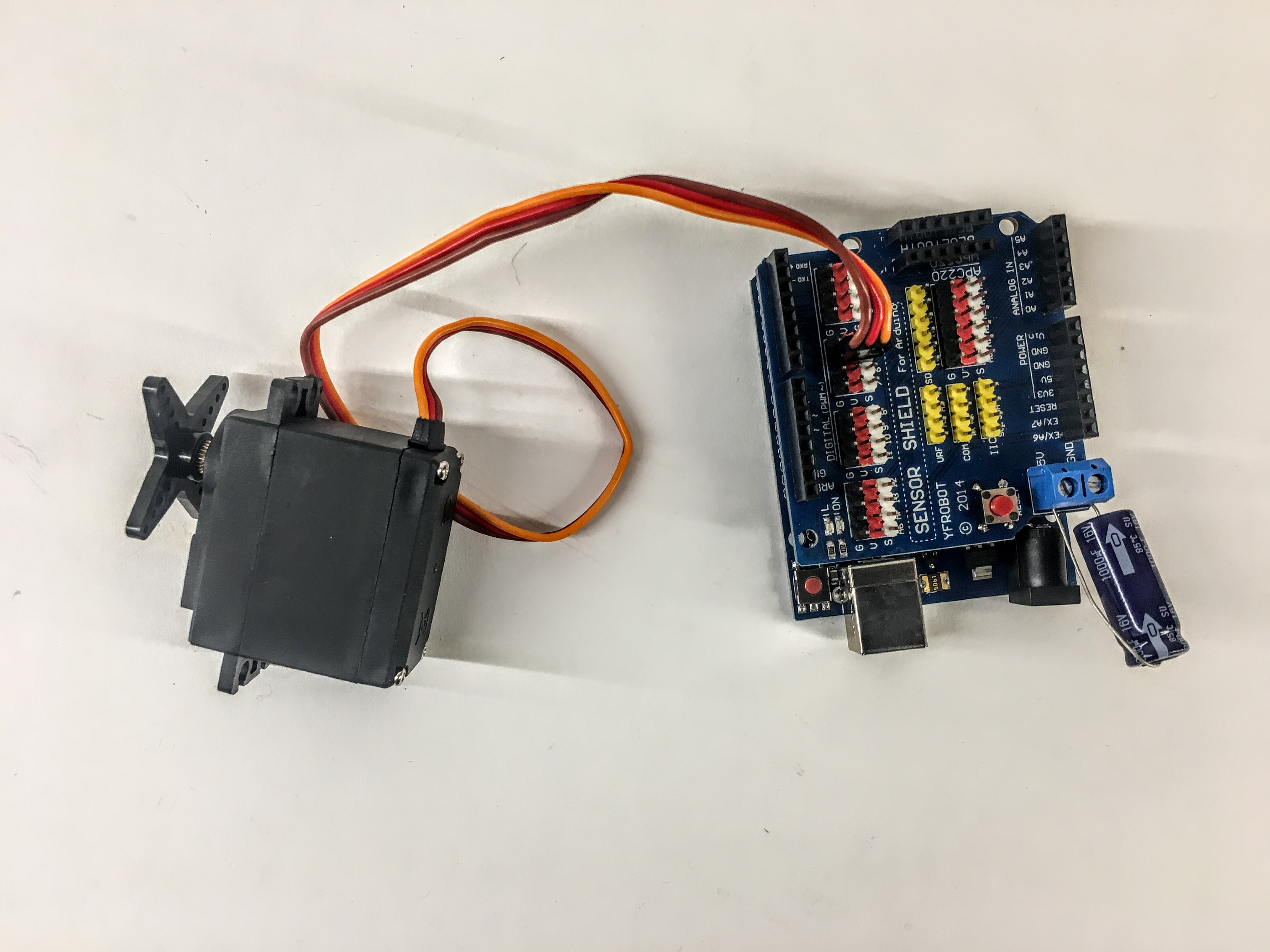``````  #include <Servo.h>

Servo myservo;  // create servo object to control a servo
// twelve servo objects can be created on most boards

int pos = 0;    // variable to store the servo position

void setup() {
myservo.attach(5);  // attaches the servo on pin 5 to the servo object
}

void loop() {

for (pos = 0; pos <= 180; pos += 1) { // goes from 0 degrees to 180 degrees
// in steps of 1 degree
myservo.write(pos);              // tell servo to go to position in variable 'pos'
delay(15);                       // waits 15ms for the servo to reach the position
}
for (pos = 180; pos >= 0; pos -= 1) { // goes from 180 degrees to 0 degrees
myservo.write(pos);              // tell servo to go to position in variable 'pos'
delay(15);                       // waits 15ms for the servo to reach the position
}
}
``````

## Joystick``````  void setup() {
Serial.begin(9600);
}

void loop() {
}
``````

## Joystick + servo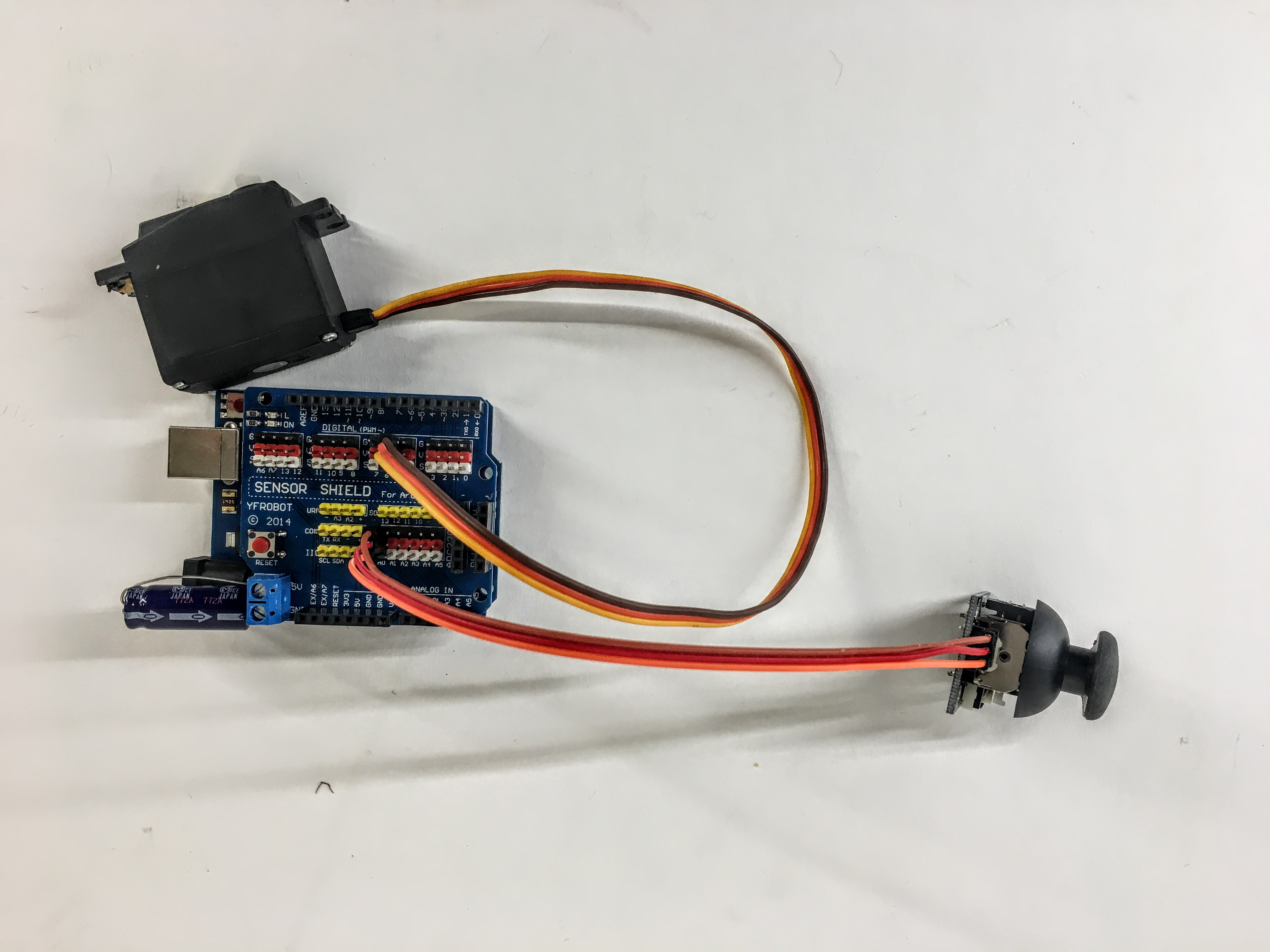``````    #include <Servo.h>

Servo myservo;

void setup() {
myservo.attach(5);  // attaches the servo on pin 5 to the servo object
}

void loop() {

}
``````

## Distance sensor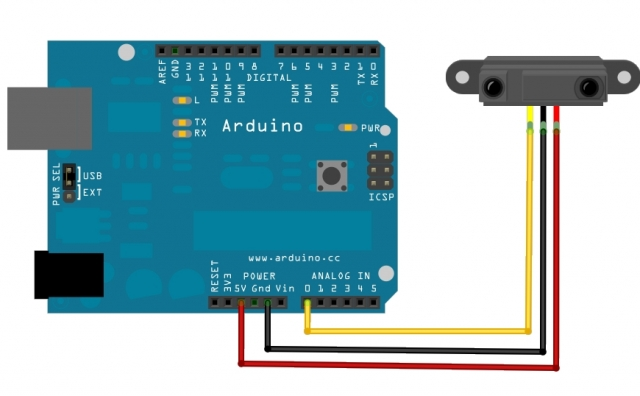One simple sensor to use is the distance sensor. This is an infrared sensor which receives values from 0-1024 relative to about 15cm to 60cm.

``````  void setup() {
Serial.begin(9600);
}

void loop() {
}
``````

## Timing without delay

Using delay is bad practice because it halts everything on the board. Instead use "millis()". This example does something every two seconds:

``````unsigned long timer = 0;
void setup()
{

}

void loop()
{
if(millis()-timer > 2000) // do something every two seconds)
{
timer = millis();
//do something here
}
}
``````

## MP3 Player

Use the MP3 player to make interactive sounds for voice commands and games.

!!! IMPORTANT - USE VERSION 1.01 for the code example below.Remember to install the library like above with neopixels: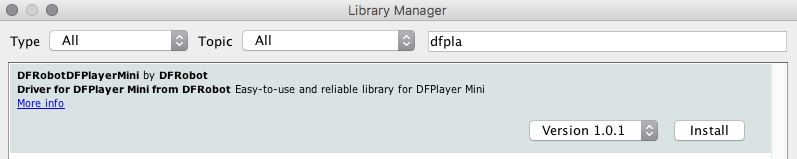This guide is the best so far

Simple sample code:

``````    #include <SoftwareSerial.h>
#include "Arduino.h"
#include <DFRobotDFPlayerMini.h>

SoftwareSerial mySoftwareSerial(10, 11); // RX, TX - mp3
DFRobotDFPlayerMini myDFPlayer;
boolean lastState = false;

void printDetail(uint8_t type, int value);
void setup() {
mySoftwareSerial.begin(9600); //for mp3 player
Serial.begin(9600);

while(!myDFPlayer.begin(mySoftwareSerial)) { //Use softwareSerial to communicate with mp3.
Serial.println(".");
delay(300);

}
myDFPlayer.volume(20); //Set volume value. From 0 to 30

pinMode(3, INPUT_PULLUP);
myDFPlayer.play(1); //Play the first mp3
}

void loop() {

}
``````

## Accelerometer

Use the accelerometer to detect movement.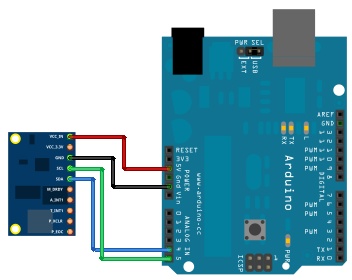``````  // Arduino code and libraries are available to download - link below the video.

// Output for viewing with Serial Oscilloscope: accX,accY,magZ // gyrX, gyrY and gyrZ are commented out

/*
Arduino     MARG GY-85
A5            SCL
A4            SDA
3.3V          VCC
GND           GND
*/

#include <Wire.h>
#include <HMC5883L.h> // HMC5883L Magnetometer Library
#include <ITG3200.h>

HMC5883L compass;
ITG3200 gyro = ITG3200();
float  gx, gy, gz;
float  gx_rate, gy_rate, gz_rate;
int ix, iy, iz;
float anglegx = 0.0, anglegy = 0.0, anglegz = 0.0;
int ax, ay, az;
int rawX, rawY, rawZ;
float X, Y, Z;
float rolldeg, pitchdeg;
int error = 0;
float aoffsetX, aoffsetY, aoffsetZ;
float goffsetX, goffsetY, goffsetZ;
unsigned long time, looptime;

void setup() {
Serial.begin(9600);
acc.powerOn();
compass = HMC5883L();
error = compass.SetScale(1.3); // Set the scale to +/- 1.3 Ga of the compass
if (error != 0) // If there is an error, print it out.
Serial.println(compass.GetErrorText(error));

// Serial.println("Setting measurement mode to continous");
error = compass.SetMeasurementMode(Measurement_Continuous); // Set the measurement mode to Continuous
if (error != 0) // If there is an error, print it out.
Serial.println(compass.GetErrorText(error));
for (int i = 0; i <= 200; i++) {
if (i == 0) {
aoffsetX = ax;
aoffsetY = ay;
aoffsetZ = az;
}
if (i > 1) {
aoffsetX = (ax + aoffsetX) / 2;
aoffsetY = (ay + aoffsetY) / 2;
aoffsetZ = (az + aoffsetZ) / 2;
}
}
for (int i = 0; i <= 200; i++) {
if (i == 0) {
goffsetX = gx;
goffsetY = gy;
goffsetZ = gz;
}
if (i > 1) {
goffsetX = (gx + goffsetX) / 2;
goffsetY = (gy + goffsetY) / 2;
goffsetZ = (gz + goffsetZ) / 2;
}
}

delay(1000);
// Serial.print("zero Calibrating...");
// gyro.zeroCalibrate(2500, 2);
// Serial.println("done.");
}

void loop() {

updateData();
// Accelerometer, Magnetometer and Gyroscope Output
Serial.print(rolldeg);
Serial.print(",");
Serial.print(pitchdeg);  // calculated angle in degrees
// Serial.print(",");

//Serial.print(anglegx);
// Serial.print(",");
//Serial.print(anglegy);
//Serial.print(",");
//Serial.println(anglegz);
//Serial.println(looptime);
}

void updateData()
{

// code fragment for Accelerometer angles (roll and pitch)
time = millis();
acc.readAccel(&ax, &ay, &az); //read the accelerometer values and store them in variables  x,y,z
rawX = ax - aoffsetX;
rawY = ay - aoffsetY;
rawZ = az  - (255 - aoffsetZ);
X = rawX / 256.00; // used for angle calculations
Y = rawY / 256.00; // used for angle calculations
Z = rawZ / 256.00; // used for angle calculations
rollrad = atan(Y / sqrt(X * X + Z * Z)); // calculated angle in radians
pitchrad = atan(X / sqrt(Y * Y + Z * Z)); // calculated angle in radians
rolldeg = 180 * (atan(Y / sqrt(X * X + Z * Z))) / PI; // calculated angle in degrees
pitchdeg = 180 * (atan(X / sqrt(Y * Y + Z * Z))) / PI; // calculated angle in degrees

// Code fragment for Magnetometer heading (yaw)
int MilliGauss_OnThe_XAxis = scaled.XAxis;// (or YAxis, or ZAxis)
float declinationAngle = 0.0457;
if (heading > 2 * PI)

// Code fragment for Gyroscope (roll, pitch, yaw)
looptime = millis() - time;
gx_rate = (gx - goffsetX) / 14.375;
gy_rate = (gy - goffsetY) / 14.375;
gz_rate = (gz - goffsetZ) / 14.375;
delay(100);
/* Serial.print(gx_rate);  // calculated angle in degrees
Serial.print(",");
Serial.print(gy_rate);
Serial.print(",");
Serial.print(gz_rate);
Serial.println(",");
*/
anglegx = anglegx + (gx_rate * looptime);
anglegy = anglegy + (gx_rate * looptime);
anglegz = anglegz + (gz_rate * looptime);

}

{
Serial.print("Raw:\t");
Serial.print(raw.XAxis);
Serial.print("   ");
Serial.print(raw.YAxis);
Serial.print("   ");
Serial.print(raw.ZAxis);
Serial.print("   \tScaled:\t");
Serial.print(scaled.XAxis);
Serial.print("   ");
Serial.print(scaled.YAxis);
Serial.print("   ");
Serial.print(scaled.ZAxis);
}
``````

Based on this instructables

## Microphone: Get the volume

You can use the little volume circuit to get the volume. I recommend you use the serial plotter in the arduino gui to see the reactions. Turn the little screw on the blue component to change the sensitivity. It should be around 500 when no sound is present.

int soundPin = A0;
float sensorValue = 0; float volume = 0;

``````    void setup ()
{
Serial.begin (9600);
}

void loop ()
{